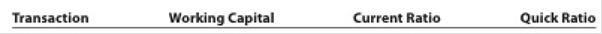# Effect of transactions on current position analysis Data pertaining to the current position of Lucroy Industries Inc. follow: Cash $800,000 Marketable securities 550,000 Accounts and notes receivable (net) 850,000 Inventories 700,000 Prepaid expenses 300,000 Accounts payable 1,200,000 Notes payable (short-term) 700,000 Accrued expenses 100,000 Instructions 1. Compute (a) the working capital, (b) the current ratio, and (c) the quick ratio. Round ratios in parts b through j to one decimal place. 2. List the following captions on a sheet of paper: Compute the working capital, the current ratio, and the quick ratio after each of the following transactions and record the results in the appropriate columns. Consider each transaction separately and assume that only that transaction affects the data given. Round to one decimal place. a. Sold marketable securities at no gain or loss,$500,000. b. Paid accounts payable, $287,500. c. Purchased goods on account,$400,000. d. Paid notes payable, $125,000. e. Declared a cash dividend,$325,000. f. Declared a common stock dividend on common stock, $150,000. g. Borrowed cash from bank on a long-term note,$1,000,000. h. Received cash on account, $75,000. i. Issued additional shares of stock for cash,$2,000,000. j. Paid cash for prepaid expenses, $200,000.BuyFind ### Accounting 27th Edition WARREN + 5 others Publisher: Cengage Learning, ISBN: 9781337272094BuyFind ### Accounting 27th Edition WARREN + 5 others Publisher: Cengage Learning, ISBN: 9781337272094 #### Solutions Chapter Section Chapter 17, Problem 17.3BPR Textbook Problem ## Effect of transactions on current position analysisData pertaining to the current position of Lucroy Industries Inc. follow: Cash$ 800,000 Marketable securities 550,000 Accounts and notes receivable (net) 850,000 Inventories 700,000 Prepaid expenses 300,000 Accounts payable 1,200,000 Notes payable (short-term) 700,000 Accrued expenses 100,000 Instructions1. Compute (a) the working capital, (b) the current ratio, and (c) the quick ratio. Round ratios in parts b through j to one decimal place.2. List the following captions on a sheet of paper:Compute the working capital, the current ratio, and the quick ratio after each of the following transactions and record the results in the appropriate columns. Consider each transaction separately and assume that only that transaction affects the data given. Round to one decimal place.a. Sold marketable securities at no gain or loss, $500,000.b. Paid accounts payable,$287,500.c. Purchased goods on account, $400,000.d. Paid notes payable,$125,000.e. Declared a cash dividend, $325,000.f. Declared a common stock dividend on common stock,$150,000.g. Borrowed cash from bank on a long-term note, $1,000,000.h. Received cash on account,$75,000.i. Issued additional shares of stock for cash, $2,000,000.j. Paid cash for prepaid expenses,$200,000.

Expert Solution

1.

To determine

Financial Ratios: Financial ratios are the metrics used to evaluate the liquidity, capabilities, profitability, and overall performance of a company.

a)

To compute: Working capital

Given info: Total current assets and current liabilities.

### Explanation of Solution

Working capital is the difference between current assets and current liabilities.

Formula:

Current ratio<

Expert Solution

2.

To determine

To compute: Working capital, Current ratio, and Quick ratio considering the given transactions.

### Want to see the full answer?

Check out a sample textbook solution.See solution

### Want to see this answer and more?

Bartleby provides explanations to thousands of textbook problems written by our experts, many with advanced degrees!

See solution